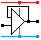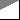Home

rOm
Quest
Glossary

Random
Page
Search
Site
Lush
Sim
Class
Subject
Images

Help
FAQ
Sign
Up
Log
In
 GCSE     Basics     Atom     BCD     Binary     Conductors     Current     Energy     >Equations<     Insulators     Maths     Power     Resistance     Safety     SI Units     Superconductors     Symbols     Transfer Characteristics     Voltage

# Basics Equations

Site for Eduqas/WJEC - Go to the AQA site.

GCSE    Basic ElectronicsQuestions 0 to 35   -->  View All

## GCSE

Resistor colour codes

 Black Brown Red Orange Yellow Green Blue Violet Grey White 0 1 2 3 4 5 6 7 8 9

Fourth Stripe Tolerance +/-        Gold = 5%        Silver = 10%

E24: 10, 11, 12, 13, 15, 16, 18, 20, 22, 24, 27, 30, 33, 36, 39, 43, 47, 51, 56, 62, 68, 75, 82, 91

Equations:   These are shown on one line with brackets, as you'd enter them into a calculator.

 P   =   V2 / R G   =   1 + RF / R1 Vout   =   Vin  R2 / ( R1 + R2) G   =   - RF / RIN ID   =   gM VGS VOUT   =   - RF ( V1 / R1 + V2 / R2 + ....) IC   =   hFE IB T   =   1.1 R C A + B   =   A . B f   =   1 / T A . B   =   A + B f   =   1.44 / ( (R1 + 2 R2 ) C ) G   =   VOUT / VIN TON / TOFF   =   ( R1 + R2 ) / R2

## AS Level

E24: 10, 11, 12, 13, 15, 16, 18, 20, 22, 24, 27, 30, 33, 36, 39, 43, 47, 51, 56, 62, 68, 75, 82, 91

 T G M k m µ n p x1012 x109 x106 x103 x10-3 x10-6 x10-9 x10-12

Equations:   These are shown on one line with brackets, as you'd enter them into a calculator.

 C   =   Q / V VRMS   =   V0 / √2 C   =   1 / ( 1 / C1 + 1 / C2 ) IRMS   =   I0 / √2 C   =   C1 C2 / ( C1 + C2 ) IC   =   hFE IB C   =   C1 + C2 ID   =   gM VGS P   =   ID2 rDSon A + A . B   =    A + B T   =   R C A . B + A   =   (B + 1)   =   A VC   =   V0 e - t / RC G   =   VOUT / VIN VC   =   V0 ( 1 - e - t / R C ) G   =   1 + RF / R1 t   =   - R C ln ( 1 - VC / V0 ) G   =   - RF / RIN t   =   - R C ln ( VC / V0 ) VOUT   =   - RF ( V1 / R1 + V2 / R2 + ....) f   ≈   1 / ( R C ) VOUT   =   VS   if   V+   >   V- f   =   1 / T VOUT   =   - VS   if   V+   <   V- T   =   1.1 R C VOUT   =   VIN tH   =   0.7 ( R1 + R2 ) C slew rate   =   Δ VOUT / Δ t tL   =   0.7 R2 C slew rate   =   2 π f Vp f   =   1.44 / ( (R1 + 2 R2 ) C ) TON / TOFF   =   ( R1 + R2 ) / R2 Vr   =   I / ( fr C )

## A Level

E24: 10, 11, 12, 13, 15, 16, 18, 20, 22, 24, 27, 30, 33, 36, 39, 43, 47, 51, 56, 62, 68, 75, 82, 91

 T G M k m µ n p x1012 x109 x106 x103 x10-3 x10-6 x10-9 x10-12

Equations:   These are shown on one line with brackets, as you'd enter them into a calculator.

 C   =   Q / V IC   =   hFE IB C   =   1 / ( 1 / C1 + 1 / C2 ) ID   =   gM VGS C   =   C1 C2 / ( C1 + C2 ) P   =   ID2 rDSon C   =   C1 + C2 A + A . B   =    A + B VRMS   =   V0 / √2 A . B + A   =   (B + 1)   =   A IRMS   =   I0 / √2 G   =   VOUT / VIN f0   =   1 / ( 2 π √ (L C) ) G   =   1 + RF / R1 RD   =   L / ( rL C ) G   =   - RF / RIN Q   =   f0 /  bandwidth   =   2 π f0 L / rL VOUT   =   - RF ( V1 / R1 + V2 / R2 + ....) NCH   =   available bandwidth / channel bandwidth VOUT   =   VS   if   V+   >   V- maximum data rate   =   2 x available bandwidth VOUT   =   - VS   if   V+   <   V- GdB   =   10 log10 ( POUT / PIN ) VOUT   =   VIN SNRdB   =   10 log10 ( PS / PN )   =   20 log10 ( VS / VN ) slew rate   =   Δ VOUT / Δ t m   =   100 ( VMAX - VMIN ) / ( VMAX + VMIN )     % slew rate   =   2 π f Vp β   =   ΔfC / f1 resolution   =   input voltage range / 2n Bandwidth   =   2 ( Δ f0 + f1 ) = 2 ( 1 + β ) f1 XC   =   1 / ( 2 π f C ) c   =   f λ XL   =   2 π f L VOUT   =   VDIFF ( RF / R1 ) Z   =   √ ( R2 + X2 ) T   =   R C Vr   =   I / ( fr C ) VC   =   V0 ( 1 - e - t / R C ) VL   ≈   VZ ( 1 + RF / R1 ) VC   =   V0 e - t / RC φ   =   tan-1 ( R / XC ) t   =   - R C ln ( 1 - VC / V0 ) fb   =   1 / ( 2 π R C ) t   =   - R C ln ( VC / V0 ) VOUT   =   VIN - 0.7 f   ≈   1 / ( R C ) VOUT   =   VIN - 3 f   =   1 / T PMAX   =   VS2 / ( 8 RL ) T   =   1.1 R C tH   =   0.7 ( R1 + R2 ) C tL   =   0.7 R2 C f   =   1.44 / ( (R1 + 2 R2 ) C ) TON / TOFF   =   ( R1 + R2 ) / R2

Subject Name     Level     Topic Name     Question Heading     First Name Last Name Class ID     User ID

 CloseEscapeX Q: qNum of last_q     Q ID: Question ID         Score: num correct/num attempts         Date Done

Question Text

image url

Help Text
Debug

• You can attempt a question as many times as you like.
• If you are logged in, your first attempt, each day, is logged.
• To improve your scores, come back on future days, log in and re-do the questions that caused you problems.
• In the grade book, you can delete your answers for a topic before re-doing the questions. Avoid deleting unless you intend re-doing the questions very soon.